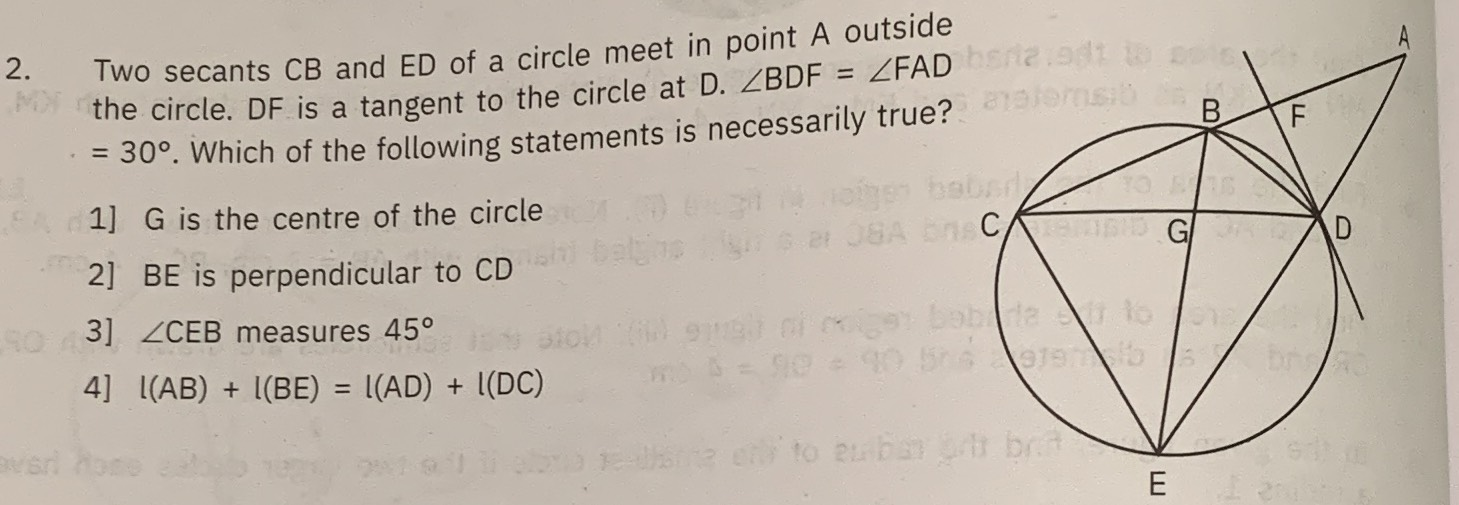### ¿Todavía tienes preguntas de matemáticas?

Pregunte a nuestros tutores expertos
Trigonometry
PreguntaTwo secants $$C B$$ and $$E D$$ of a circle meet in point $$A$$ outside the circle. DF is a tangent to the circle at D. $$\angle B D F = \angle F A D$$

$$= 30 ^ { \circ } .$$ Which of the following statements is necessarily true? 1] $$G$$ is the centre of the circle 2] $$B E$$ is perpendicular to $$C D$$ 3] $$\angle C E B$$ measures $$45 ^ { \circ }$$

4] $$l ( A B ) + I ( B E ) = I ( A D ) + l ( D C )$$